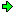World!Of
NumbersWON plate
188 |

[ January 16, 2014 ]
Gathering prime factors of nine- and pandigitals
and extracting statistical information from the data

How many different primes divide all the ninedigitals ?
How many different primes divide all the pandigitals ?
(each digit from set [1-9] or [0-9] appears just once)

What is the largest prime factor in each case ?

What prime factor(s) has the least frequency of appearing ?

How many palindromic prime factors are there in both cases ?

List of smallest and largest nine-/pandigital 3k * semiprimes ?

 To start with I give the smallest and largest nine- and pandigital prime factorization. 123456789 = 32 x 3607 x 3803 987654321 = 32 x 172 x 379721 yielding already this subset {... ,3,...,17,...,3607,...,3803,...,379721,... } and 1023456789 = 34 x 2221 x 5689 9876543210 = 2 x 32 x 5 x 172 x 379721 yielding already this subset {2,3,5,...,17,...,2221,...,5689,...,379721,... }Some related sources

A178775 Smallest prime factors of zerofull restricted pandigital numbers.
A204532 Largest prime factors of zerofull restricted pandigital numbers A050278.

A216203 Smallest prime that does not divide at least one n-digit zeroless pandigital number.
A228253 Smallest prime that does not divide at least one n-digit pandigital number.

Puzzle 259 Not dividing any pandigital.
Puzzle 926 Pandigital and prime numbers.QuestionsAnswers by Alexandru Petrescu [ April 4, 2021 ]
NinedigitalsPandigitals
How many different primes divide
all the nine- and pandigitals ?
36419252702
What is the largest prime factor ? 109739359  * 9 = 987654231 1097393447  * 9 = 9876541023
How many palindromic prime factors
appear ?
23104
Can you list them ?2, 3, 5, 7, 11, 101, 131, 151, 181, 191,
313, 353, 373, 383, 727, 757, 787, 797,
919, 929, 1093901, 9561659, 9583859
The largest palindromic prime factor ? 9583859  * 27 = 258764193 955141559  * 9 = 8596274031
Prime factors having the least
frequency of appearing ?
There are many 9digits prime factors,
when multiplied by 9 provide a
unique ninedigital !
There are many 10digits prime factors,
when multiplied by 10 provide a
unique pandigital !
Numbers = 3k * p * q ;
(3, p, q distinct primes)
Semiprimes topictotal numbers [version A]
75330565732
Smallest
123456789 = 32 *  3607  *  3803 1023456789 = 34 *  2221  *  5689
Largest
987654123 = 32 *  109  *  1006783 9876542103 = 32 *  53  *  20705539
Numbers = 3k * p ;
(3, p distinct primes)
Semiprimes topictotal numbers [version B]
35319249804
Smallest
123458679 = 32 *  13717631 1023456879 = 32 *  113717431
Largest
987654231 = 32 *  109739359 9876542301 = 38 *  1505341A000188Prime Curios!Prime PuzzleWikipedia 188Le nombre 188```

```

[TOP OF PAGE]

Patrick De Geest - Belgium- Short Bio - Some Pictures